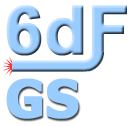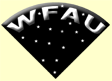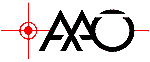* DR3 now active *
 Menu | SQL | XmatchIFA     ROE

### FITS file - VR spectrum extension

Combined spectrum
```XTENSION= 'IMAGE   '           / IMAGE extension
BITPIX  =                  -32 / number of bits per data pixel
NAXIS   =                    2 / number of data axes
NAXIS1  =                 2244 / length of data axis 1
NAXIS2  =                    4 / length of data axis 2
PCOUNT  =                    0 / required keyword; must = 0
GCOUNT  =                    1 / required keyword; must = 1
CRVAL1  =             3932.253 / Co-ordinate value of axis 1
CDELT1  =             1.633849 / Co-ordinate increment along axis 1
CRPIX1  =                 1.00 / Reference pixel along axis 1
CTYPE1  = 'LINEAR  '           / coordinate system
CUNIT1  = 'Angstroms'          / Units for axis 1
CRVAL2  =                 1.00 / Co-ordinate value of axis 2
CDELT2  =                 1.00 / Co-ordinate increment along axis 2
CRPIX2  =                 1.00 / Reference pixel along axis 1
CTYPE2  = 'LINEAR  '           / coordinate system
CROTA2  =                 0.00 / DEPRECATED - rotation of axis 2
CD1_1   =             1.633849 / Co-ordinate transformation matrix
CD1_2   =                 0.00 / Co-ordinate transformation matrix
CD2_2   =                 1.00 / Co-ordinate transformation matrix
CD2_1   =                 0.00 / Co-ordinate transformation matrix
PC001001=                 1.00 / Axis rotation matrix
PC001002=                 0.00 / Axis rotation matrix
PC002001=                 0.00 / Axis rotation matrix
PC002002=                 1.00 / Axis rotation matrix
EXTNAME = 'SPECTRUM VR'        / VR combined spectrum
NAME_V  = 'g0000531-300714'    / Observed object name V frame
TARGET  = 'g0000531-300714'    / matching name in config file
OBSRA   =           0.22112500 / Observed RA
OBSDEC  =         -30.12055556 / Observed DEC
MATCH_DR=               0.0006 / position match to TARGET in arcsec
Z       =             0.039750 / raw measured redshift
Z_HELIO =             0.039813 / heliocentric corrected redshift
QUALITY =                    1 / redshift measurement quality
ABEMMA  =                    1 / redshift source abs1 emm2 man3
NMBEST  =                    1 / number emission lines for best z_em
NGOOD   =                    1 / number of good emission lines
Z_EMI   =             0.115852 / emission redshift
Q_Z_EMI =                    0 / emission redshift quality
KBESTR  =                    4 / x-correlation template
R_CRCOR =             3.920000 / x-correlation peak
Z_ABS   =             0.039746 / x-correlation redshift
Q_Z_ABS =                    3 / x-correlation quality
Q_FINAL =                    3 / Suggested quality for redshift
IALTER  =                    0 / IALTER=1 if automatic z altered
Z_COMM  = '        '           / runz comment
END
```

Home | Intro | Schema | Access | FITS files

WFAU, Institute for Astronomy,
Royal Observatory, Blackford Hill
Edinburgh, EH9 3HJ, UK
Tel +44 131 668 8356 (office)
or +44 131 668 8100 (switchboard)

6dfdata@aao.gov.au
26/8/2008